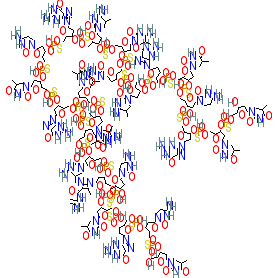#### GtoPdb Ligand ID: 9843

Synonyms: CpG 7909 | CpG7909 | PF 3512676 | PF-3512676 | ProMune | VaxImmune
Compound class: Synthetic organic
Comment: Agatolimod is synthetic CpG oligodeoxynucleotide that acts as a TLR9 agonist . We show the parent molecule, whereas the USAN specifies agatolimod sodium (PubChem CID 56841789), and the synonyms we list may also refer to the sodium congener. Its nucleotide sequence is d(P-thio)(T-C-G-T-C-G-T-T-T-T-G-T-C-G-T-T-T-T-G-T-C-G-T-T).
2D StructurePhysico-chemical PropertiesHydrogen bond acceptors 165 Hydrogen bond donors 55 Rotatable bonds 143 Topological polar surface area 3684.42 Molecular weight 7701.8 XLogP 18.21 No. Lipinski's rules broken 4
SMILES / InChI / InChIKeyCanonical SMILES OCC1OC(CC1OP(=S)(OCC1OC(CC1OP(=S)(OCC1OC(CC1OP(=S)(OCC1OC(CC1OP(=S)(OCC1OC(CC1OP(=S)(OCC1OC(CC1OP(=S)(OCC1OC(CC1OP(=S)(OCC1OC(CC1OP(=S)(OCC1OC(CC1OP(=S)(OCC1OC(CC1OP(=S)(OCC1OC(CC1OP(=S)(OCC1OC(CC1OP(=S)(OCC1OC(CC1OP(=S)(OCC1OC(CC1OP(=S)(OCC1OC(CC1OP(=S)(OCC1OC(CC1OP(=S)(OCC1OC(CC1OP(=S)(OCC1OC(CC1OP(=S)(OCC1OC(CC1OP(=S)(OCC1OC(CC1OP(=S)(OCC1OC(CC1OP(=S)(OCC1OC(CC1OP(=S)(OCC1OC(CC1OP(=S)(OCC1OC(CC1O)n1cc(C)c(=O)[nH]c1=O)O)n1cc(C)c(=O)[nH]c1=O)O)n1cnc2c1nc(N)[nH]c2=O)O)n1ccc(nc1=O)N)O)n1cc(C)c(=O)[nH]c1=O)O)n1cnc2c1nc(N)[nH]c2=O)O)n1cc(C)c(nc1=O)N)O)n1cc(C)c(nc1=O)N)O)n1cc(C)c(nc1=O)N)O)n1cc(C)c(nc1=O)N)O)n1cnc2c1nc(N)[nH]c2=O)O)n1ccc(nc1=O)N)O)n1cc(C)c(=O)[nH]c1=O)O)n1cnc2c1nc(N)[nH]c2=O)O)n1cc(C)c(=O)[nH]c1=O)O)n1cc(C)c(=O)[nH]c1=O)O)n1cc(C)c(nc1=O)N)O)n1cc(C)c(nc1=O)N)O)n1cnc(c1C)C(=O)NC(=C)N)O)n1ccc(nc1=O)N)O)n1cc(C)c(=O)[nH]c1=O)O)n1cnc2c1nc(N)[nH]c2=O)O)n1ccc(nc1=O)N)O)n1cc(C)c(=O)[nH]c1=O Isomeric SMILES OCC1OC(CC1OP(=S)(OCC1OC(CC1OP(=S)(OCC1OC(CC1OP(=S)(OCC1OC(CC1OP(=S)(OCC1OC(CC1OP(=S)(OCC1OC(CC1OP(=S)(OCC1OC(CC1OP(=S)(OCC1OC(CC1OP(=S)(OCC1OC(CC1OP(=S)(OCC1OC(CC1OP(=S)(OCC1OC(CC1OP(=S)(OCC1OC(CC1OP(=S)(OCC1OC(CC1OP(=S)(OCC1OC(CC1OP(=S)(OCC1OC(CC1OP(=S)(OCC1OC(CC1OP(=S)(OCC1OC(CC1OP(=S)(OCC1OC(CC1OP(=S)(OCC1OC(CC1OP(=S)(OCC1OC(CC1OP(=S)(OCC1OC(CC1OP(=S)(OCC1OC(CC1OP(=S)(OCC1OC(CC1OP(=S)(OCC1OC(CC1O)n1cc(C)c(=O)[nH]c1=O)O)n1cc(C)c(=O)[nH]c1=O)O)n1cnc2c1nc(N)[nH]c2=O)O)n1ccc(nc1=O)N)O)n1cc(C)c(=O)[nH]c1=O)O)n1cnc2c1nc(N)[nH]c2=O)O)n1cc(C)c(nc1=O)N)O)n1cc(C)c(nc1=O)N)O)n1cc(C)c(nc1=O)N)O)n1cc(C)c(nc1=O)N)O)n1cnc2c1nc(N)[nH]c2=O)O)n1ccc(nc1=O)N)O)n1cc(C)c(=O)[nH]c1=O)O)n1cnc2c1nc(N)[nH]c2=O)O)n1cc(C)c(=O)[nH]c1=O)O)n1cc(C)c(=O)[nH]c1=O)O)n1cc(C)c(nc1=O)N)O)n1cc(C)c(nc1=O)N)O)n1cnc(c1C)C(=O)NC(=C)N)O)n1ccc(nc1=O)N)O)n1cc(C)c(=O)[nH]c1=O)O)n1cnc2c1nc(N)[nH]c2=O)O)n1ccc(nc1=O)N)O)n1cc(C)c(=O)[nH]c1=O InChI Key GUVMFDICMFQHSZ-UHFFFAOYSA-N
 References 1. Millward M, Underhill C, Lobb S, McBurnie J, Meech SJ, Gomez-Navarro J, Marshall MA, Huang B, Mather CB. (2013) Phase I study of tremelimumab (CP-675 206) plus PF-3512676 (CPG 7909) in patients with melanoma or advanced solid tumours. Br J Cancer, 108 (10): 1998-2004. [PMID:23652314] 2. Thompson JA, Kuzel T, Drucker BJ, Urba WJ, Bukowski RM. (2009) Safety and efficacy of PF-3512676 for the treatment of stage IV renal cell carcinoma: an open-label, multicenter phase I/II study. Clin Genitourin Cancer, 7 (3): E58-65. [PMID:19815483] 3. (2006) CpG 7909: PF 3512676, PF-3512676. Drugs R D, 7 (5): 312-6. [PMID:16922592]